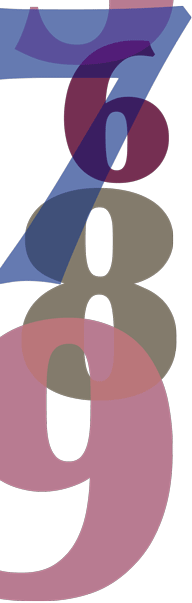# Year 7

## Number and Algebra

### Rounding decimals

With many decimal representations of numbers, the last few decimal places may have no practical value. For money, dollars and cents need two decimal places for ordinary transactions. However, supermarkets and other retail outlets round to the nearest five cents when a consumer is paying with cash because one-cent and two-cent coins are no longer legal tender.

With measurement, if 3.241 592 7 represents the number of kilometres between two farmyard gates, then the final digit, 7, represents $$\dfrac{7}{10}$$ of a millimetre, here a completely insignificant and irrelevant amount. In this example, the distance correct to the nearest metre would be the most appropriate, so we would say the distance was 3.242 km. This process is called rounding.